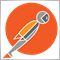# Anyone want to take a crack at this?43

```int OnInit()
{
//---
double ticksize = MarketInfo(Symbol(), MODE_TICKSIZE);
if (ticksize == 0.00001 || ticksize == 0.001)
pips = ticksize*10;
else pips=ticksize;

Alert("Ticksize = ",ticksize," Pips = ",pips);

if(bias == false)//*****************SELL BIAS
{
BHPLS=((SOP-LowerLimit)/(ProfitTarget*pips)+1);// bottom half price levels total..SO IF SOP IS .7273 (below open price of
//the backtest first bar) then ((.7273-.60)/(40*.0001)+1)=32.825 so 32..and this is correct according to journal
BPL=(SOP-((BHPLS-1)*ProfitTarget*pips)); // bottom price level..SO IF BHPLS=32 THEN
//(.7273-(32-1)*.004)=.6033..and this is correct according to journal
Alert("BHPLS = ",BHPLS," BPL = ",BPL);
PLT=((UpperLimit-BPL)/(ProfitTarget*pips))+1; //price levels total =((.85-.6033)/.004)+1=62.675=62..this is also correct
Alert ("PLT = ",PLT); //price levels total = 62

for(i=0; i<BHPLS; i++)
{
PriceLevel=(i*ProfitTarget*pips)+BPL; //when i=0 and BPL =.6033 then Pricelevel is
// (0*40*.0001)+.6033 = .6033
MagicNumber=PriceLevel/pips;// MagicNumber would be .6033/.0001 so no idea how it is coming up 6032

if ( OrderSend(Symbol(),OP_SELLSTOP,Lots,PriceLevel,5*pips,0,0,NULL,MagicNumber,0,White) == false)
Alert("Order SellStop failed, error # ", GetLastError() );
else
{
Alert("MagicNum = ",MagicNumber," PriceLev = ",PriceLevel);
}

```

Hi All,

Anyone want to take a crack at this?

This code will ask for inputs, so in this case the following were entered

2019.03.11 11:56:18.393 2016.01.04 00:00:00  12th March inputs: Lots=0.01; ProfitTarget=40; UpperLimit=0.85; LowerLimit=0.6; bias=0; StopTrigger=80; SOP=0.7273;

for some reason which for the life of me I cannot work out, some of my orders (these are pending orders by the way) the magic number will come out 1 digit different to the pricelevel, and for other orders it comes out the same

As can be seen from this screenshot of my Journal (attached), some sellstops have different magicnumbers to the pricelevels, others are the same

i am stumped on this one

btw, this is on the AUDUSD chart

Files:
Capture.PNG 76 kb2392

That defeats the propose of magic numbers.43

Hi nicholi,

Yes agreed, it is not the intent of the magic numbers to be used like this, but I am unable to find any other way to give each order a unique number that I can refer back to so that number can be used to set the orderopenprice.

for instance, if a position is filled under slippage (so instead of .7273 it gets filled at .7275) and then if that position goes into profit and I set say a stop at breakeven or higher, I want to reopen a pending order at .7273 and not at the price it was actually opened at (in this case .7275). Hence the reason I am giving each order a magic number that is calculated at the init stage.

if there was another way I could give each order a unique number then that might solve my problem